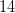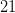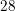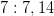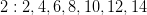SSAT Upper Level Math : How to find the lowest / least common denominator

Example Questions

2 Next →

Example Question #11 : How To Find The Lowest / Least Common Denominator

Find the least common denominator for the fractions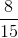and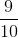.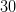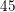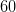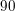Explanation:

To find the least common denominator, list out the multiples for each denominator then choose the lowest one that appears in both sets.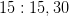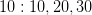Example Question #12 : How To Find The Lowest / Least Common Denominator

Find the least common denominator for the fractions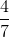and